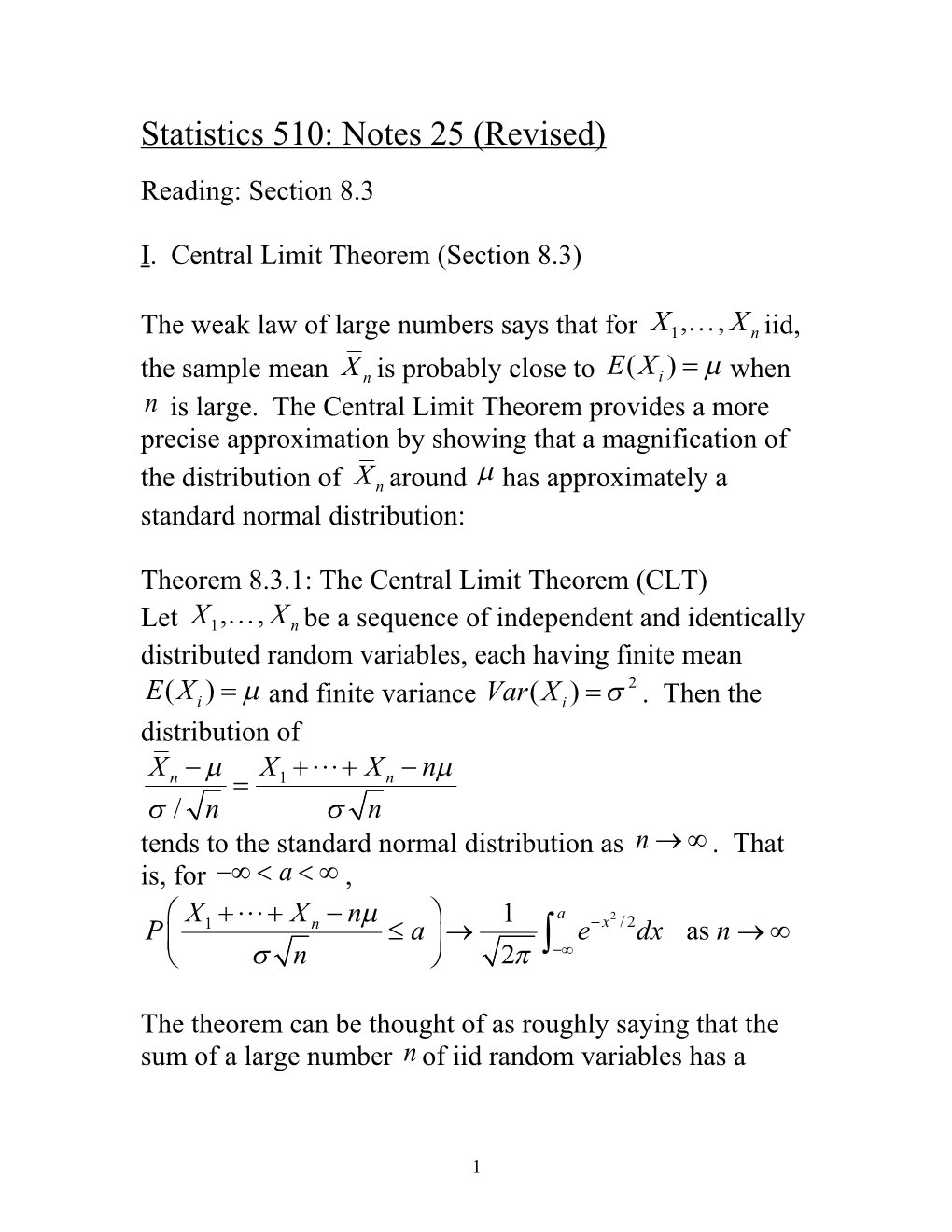# Statistics 510: Notes 25 (Revised)Statistics 510: Notes 25 (Revised)

I. Central Limit Theorem (Section 8.3)

The weak law of large numbers says that for iid, the sample mean is probably close to when is large. The Central Limit Theorem provides a more precise approximation by showing that a magnification of the distribution of around has approximately a standard normal distribution:

Theorem 8.3.1: The Central Limit Theorem (CLT)

Let be a sequence of independent and identically distributed random variables, each having finite mean and finite variance . Then the distribution of

tends to the standard normal distribution as . That is, for ,

The theorem can be thought of as roughly saying that the sum of a large number of iid random variables has a distribution that is approximately normal with mean and variance . By writing

we see that the CLT says that the sample mean has a approximately a normal distribution with mean and variance .

The CLT is a remarkable result – only assuming that a sequence of iid random variables have a finite mean and variance, the central limit theorem shows that the mean of the sequence, suitably standardized, always converges to having a standard normal distribution.

Note: The normal approximation to the binomial distribution from Section 5.4.1 is a special case of the central limit theorem.

Before examining the proof, we look at a few applications.

Example 1: Do you believe a friend who claims to have tossed heads 5,250 times in 10,000 tosses of a fair coin?

Example 2: Bill makes 100 check transactions between receiving two consecutive bank statements. Rather than subtract each amount exactly, he rounds each entry off to the nearest dollar. Let denote the round-off error associated with the th transaction [it can be assumed that has a uniform distribution over the interval (-0.5,0.5)]. Use the central limit theorm to approximate that Bill’s total accumulated error (either positive or negative) after 100 transactions exceeds \$5.

Proof of the Central Limit Theorem

We know from Chapter 7.7 that a distribution function is uniquely determined by its moment generating function. The following lemmastates that this unique determination holds for limits as well (see W. Feller, An Introduction to Probability Theory and Its Applications, Volume II, 1971, for the proof)

Lemma 8.3.1: Let be a sequence of random variables having distribution functions and moment generating functions ; and let Z be a random variable having distribution function and moment generating function . If for all t, then for all t at which is continuous.

If we let Z be a standard normal random variable, then, as , it follows from Lemma 8.3.1, that if as , then as . The central limit theorem is proved by showing that the moment generating functions of

converge to as .

Proof of central limit theorem: We shall prove the theorem under the assumption that the moment generating function of the exists and is finite. Let . Note that .

are independent so that the moment generating function of is

and the moment generating function of

is

.

Let . To prove the theorem, we much show that as or equivalently that

as .

To show this, note thatNote further thatThus,

,

which proves that as and hence that

by Lemma 8.3.1.

How large does need to be for the CLT to provide a good approximation?

For practical purposes, especially for statistics, the limiting result in the CLT is not of primary interest. Statisticians are more interested in its use as an approximation with finite values of n. It is impossible to give a concise and definitive statement of how good the approximation is, but some general guidelines are available, and examining special cases can give insight. How fast the approximation becomes good depends on the distribution of the ’s. If the distribution is fairly symmetric and has tails that die off rapidly, the approximation becomes good for relatively small values of n. If the distribution is very skewed or if the tails die down very slowly, a larger value of n is needed for a good approximation.

Example 3: Since the uniform distribution on (0,1) has mean and variance , the sum of 12 uniform random variables, minus 6, has mean 0 and variance 1. The central limit theorem says that the distribution of this sum is approximately standard normal and in fact the distribution is quite close to the standard normal. In fact, before better algorithms were developed, the sum of 12 uniform random variables minus 6 was commonly used in computers for generating normal random variables from uniform ones.Example 4: Dice rolls. Let be independent and identically distributed rolls of a die that has probability mass function

The charts attached to this note show histograms of the probability distribution for the sums for rolls of the die for

(1) the unbiased die with the symmetrical distribution

(2) the biased die with the asymmetrical distribution

It is quite apparent that for both distributions (1) and (2) of the die, the distribution of the sums is approximately a normal (bell-shaped) distribution by , but the sums take on an approximately normal distribution earlier for the symmetric distribution (1) than for the asymmetric distribution (2).

The speed of convergence of the distribution of the sample mean to its limiting distribution for different distributions is also illustrated by the applet at .

1